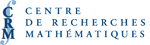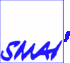# PARTENAIRESAnalyse asymptotique de motifs localisés dans les EDPs (SCMAI)
Org: David Iron et Theodore Kolokolnikov (Dalhousie)
[PDF]

GUILLEMETTE CHAPUISAT, ENS Cachan, 61, av. du Président Wilson, 94235 Cachan Cedex, France
Model of spreading depression and existence of travelling front
[PDF]

Spreading depression is a transient depolarization of neurons that spreads slowly through a part of the brain during stroke, epilepsy or migraine with aura. They have been observed and studied in most animal species for more than 50 years, but their existence in the human brain is still discussed. Mathematical models of spreading depressions have been established; they are linked to a reaction-diffusion mechanism. After some numerical experiments, we have made the following hypothesis: The mechanisms that trigger spreading depressions are the same in the human brain as in the rodent brain, but the morphology of the human brain could explain the non-observation of these waves.

Hence I have studied the following equation:

 ¶t u - \triangle u = lu (u-q)(1-u) 1|z| < R - au 1|z| ³ R
where (x,z) Î RN is the space variable.

I have proved that if R is small enough, there is no travelling front solution of these equation. And if R is large enough, there exists a travelling front in the x-direction. This result is obtained by studying the energy of a solution with special initial conditions in several travelling referentials.

DAVID IRON, Dalhousie University, Department of Mathematics and Statistics, Halifax, NS B3H 3J5
Stability of curved interfaces to the two dimensional perturbed Allen-Cahn equations
[PDF]

We consider equilibrium solutions to a perturbed Allen-Cahn model in bounded 2-dimensional domains that have the form of a curved interface. Using singular perturbation techniques, we fully characterize the stability of such an equilibrium in terms of a certain geometric eigenvalue problem, and give a simple geometric interpretation of our stability results. Full numerical computations of the associated two-dimensional eigenvalue problem are shown to be in excellent agreement with the analytical predictions.

THEODORE KOLOKOLNIKOV, Dalhousie
Ring solutions in RN and smoke-ring (vortex) solutions in R3 for Gierer-Meinhardt Model
[PDF]

We consider the classical Gierer-Meinhardt Model in N dimensions,

 e2 Du-u+ up vq = 0,     Dv-v + um vs = 0
where e is assumed to be small.

A ring-type solution in RN is a solution that concentrates on the surface of an N-sphere as e® 0. On the other hand, a smoke-ring or vortex solution in R3 is a solution that concentrates on the perimeter of a two-dimensional circle.

For ring solutions, assume

 0 < p-1 q < a¥ if N = 2,     and     0 < p-1 q < 1 if N ³ 3
where a¥ > 1 whose numerical value is a¥=1.06119. We prove that there exists a unique Ra > 0 such that for R Î (Ra,+¥], there is a ring-type solution inside the ball of radius R (R=+¥ corresponds to RN case), that concentrates on the surface of a ball of radius 0 < r0 < R. Moreover depending on parameter values, there are either exactly one or two choices for r0.

For smoke-ring solutions, we study the case when the domain is all of R3. We then show that a smoke-ring solution concentrates on a circle whose radius is precisely r0 = 0.43385.

The analysis of ring solutions relies heavily on manipulation of Bessel functions. The analysis for smoke-ring solutions involves a deep expansion of a certain singular integral.

This is a joint work with Juncheng Wei (rings) and with Xiaofeng Ren (smoke-rings).

Stationary spot solutions in an activator-inhibitor system
[PDF]

In this talk I will discuss the existence of spot solutions in a FitzHugh-Nagumo type system. These solutions turn out to be stable thanks to a delicate balance between the tendency of the system to locally minimize its energy and the curve shortening effect.

This is a joint work with Xinfu Chen.

YASUMASA NISHIURA, RIES, Hokkaido University, N12, W6, Kita-ku, Sapporo, Japan
Dynamics of Particle Patterns in Dissipative Systems
[PDF]

Particle patterns mean any spatially localized structures sustained by the balance between inflow and outflow of energy/material which arise in the form of chemical blob, discharge pattern, morphological spot, and binary convection cell. These are modeled by typically three-component reaction diffusion systems or a couple of complex GL equations with concentration field. They collide with each other, interact with defects and experience large deformation and/or basin-switching of dynamics in the form of merging, annihilation, rebound, and pinning to the defect created by heterogeneities. A new viewpoint based on a network of hidden saddles is presented to reveal the skeletal structure of those complex transient dynamics.

XIAOFENG REN, George Washington University
Ideal and Defective Solutions to a Free Boundary Problem from Block Copolymer Morphology
[PDF]

The Ohta-Kawasaki density functional theory of diblock copolymers gives rise to a nonlocal free boundary problem. In a proper parameter range an equilibrium pattern of many droplets is proved to exist in a general planar domain. A sub-range is identified where the multiple droplet pattern is stable. A defective ring pattern solution is also found. The importance of the the resonance condition is carefully studied.

MICHAEL WARD, Dept. of Mathematics, UBC, Vancouver, V6T 1Z2
Self-Replicating Spots for Reaction-Diffusion Models in Two Space Dimensions
[PDF]

We analyze the dynamical behavior of multi-spot solutions in a two-dimensional domain W for certain two-component reaction-diffusion models, including the Gray-Scott model, in the singularly perturbed limit of small diffusivity e for one of the two components. A formal asymptotic analysis, which has the effect of summing infinite logarithimic series in powers of -1/loge, is used to derive an differential algebraic system of ODE's characterizing the slow dynamics of the spot locations. By numerically examining the stability thresholds for a single spot solution, a specific and simple criterion is formulated to theoretically predict the initiation spot-replication events. The analytical theory is compared with full numerical results.

REBECCA WHITE, Dalhousie